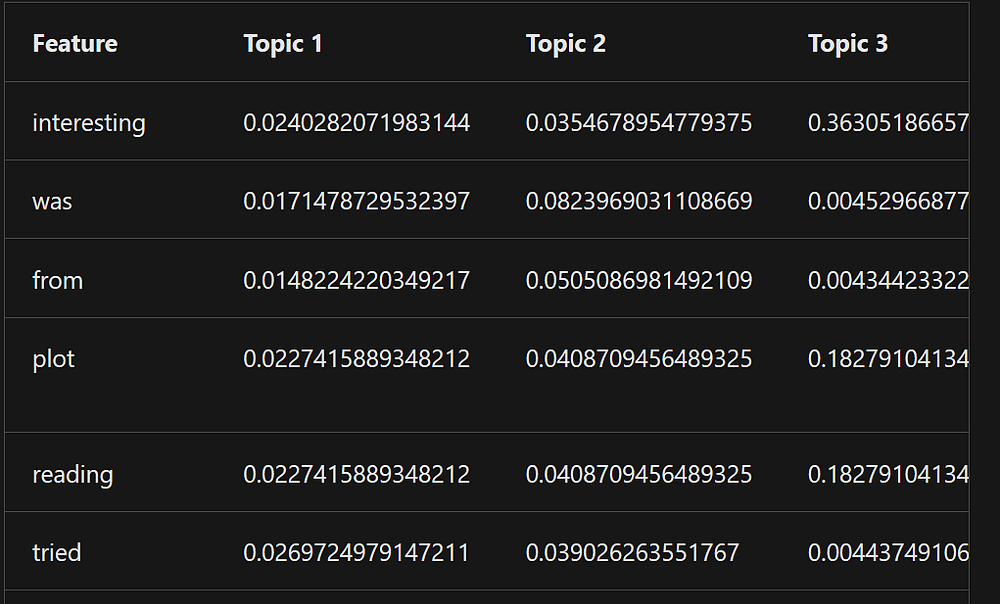Search
• Alibek Jakupov

# LDA on Azure ML Studio: Harder, Better, Faster, Stronger

Updated: May 17, 2020As topic modeling has increasingly attracted interest from researchers there exists plenty of algorithms that produce a distribution over words for each latent topic (a linguistic one) and a distribution over latent topics for each document. The list consists of explicit Dirichlet Allocation that incorporates a preexisting distribution based on Wikipedia; Concept-topic model (CTM) where a multinomial distribution is placed over known concepts with associated word sets; Non-negative Matrix Factorization that, unlike the others, does not rely on probabilistic graphical modeling and factors high-dimensional vectors into a low-dimensionally representation. However most of them are often based off Latent Dirichlet Allocation (LDA) which is a state-of-the-art method for generating topics.

All the developers working directly or indirectly with natural language are definitely familiar with topic modeling, especially with Latent Dirichlet Allocation. This magic tool, created by David Blei, allows to bring some order into your unstructured textual data and represents all the corpus (collection of documents) as a combination of topics, where each document belongs to a given topic with a certain probability. This algorithm has been used for document summarization, word sense discrimination, sentiment analysis, information retrieval and image labeling.

In LDA each document in the corpus is represented as a multinomial distribution over topics. Each topic is represented as the multinomial distribution over words. Based on the likelihood it is possible to claim that only a small number of words are important. In this case the model simultaneously learns the topics by iteratively sampling topic assignment to every word in every document (in other words calculation of distribution over distributions), using the Gibbs sampling update.

As it has been mentioned above every topic is a multinomial distribution over terms. Consequently, a standard way of interpreting a topic is extracting top terms with the highest marginal probability (a probability that the terms belongs to a given topic). However, for tasks where the topics distributions are provided to humans as a 1rst-order output, it may be difficult to interpret the rich statistical information encoded in the topics.

In r there is an excellent tm package (which is already pre-installed on AML virtual machine) that contains the LDA facility:

```library(tm)
library(topicmodels)
corp <- Corpus(VectorSource(c(“your documents frame”) ))
dtm <- DocumentTermMatrix(corp)
# Number of topics
k <- 10
# Run LDA using Gibbs sampling
ldaOut <-LDA(dtm,k, method="Gibbs")
ldaOutTerms <- as.data.frame(terms(ldaOut,20))```

This code allows you to see the topics as this multinomial distribution, like in the following image (taken from David Blei’s research paper - M. I. J. David M. Blei, Andrew Y. Ng. Latent dirichlet allocation. Journal of Machine Learning Research, 3, 2003 )And add the following line to see the gamma topics distribution

However, if you want to see only the top topics per document, which makes sense, as in the real world a document is related only to a limited number of topics, add the following code:

```# setting first row as column names
# capitalizing fisrt letter of the column names
# Now for each doc, find just the top-ranked topic
toptopics\$topic <- sapply(toptopics\$topic,function (x) paste(unlist(x), collapse = ';'))
toptopics\$document <- sapply(toptopics\$document,function (x) unlist(x))```

If you want to output your R script module, then just set the ldaOutTerms to the maml output port.

Simple and beautiful, right? However, it takes ages to run the LDA on a huge corpus even on the local machine to say nothing of the virtual environment, where it may take several hours and crash.

Another solution may be using Vowpal Wabbit module, which is memory friendly and is very easy to use. According to Microsoft Docs (https://docs.microsoft.com/en-us/azure/machine-learning/studio-module-reference/latent-dirichlet-allocation):

This module takes a column of text, and generates these outputs:
The source text, together with a score for each category
A feature matrix, containing extracted terms and coefficients for each category
A transformation, which you can save and reapply to new text used as input
Because this module uses the Vowpal Wabbit library, it is very fast.

Here is the list of all the manipulations to set your clusterization experiment up and running.

2. Provide a dataset with a textual column as a target column

3. Set Number of topics to model, (integer between 1 and 1000). By default, 5 topics are created.

4. Specify the maximum length of N-grams generated during hashing. By default unigrams and bigrams are generated

5. Select the Normalize option to converting output values to probabilities. We will use this later

After you have followed all the steps the module output represents all the documents with their most relevant topics and all the terms with their topics.

However, if we look at the second output…It does not at all look like our r script output. Nevertheless, the output is saved as a dataframe, thus we could try applying some transformation and obtain our top terms.

This time we will use Python scripting module.

```import pandas as pd

NUM_TOP_TERMS = 10

# The entry point function can contain up to two input arguments:
#   Param<dataframe1>: a pandas.DataFrame representing gamma distribution of terms in LDA model
def azureml_main(dataframe1, dataframe2=None):
# output dataframe
output = pd.DataFrame()
# iterate over columns
for column_name in list(dataframe1):
# except the first one named 'Feature'
if str(column_name) != 'Feature':
print(column_name)
# temp dataframe contain the current column and features
# an array containing top terms
top_terms = list(temp['Feature'])
print(top_terms)
output[column_name] = top_terms
# Return value must be of a sequence of pandas.DataFrame
return output```

This will convert the output into our usual top terms matrix.

Now we can run our LDA in an extremely fast and efficient manner.

Enjoy!